Tamilnadu State Board New Syllabus Samacheer Kalvi 11th Chemistry Guide Pdf Chapter 6 Gaseous State Text Book Back Questions and Answers, Notes.

## Tamilnadu Samacheer Kalvi 11th Chemistry Solutions Chapter 6 Gaseous State

### 11th Chemistry Guide Gaseous State Text Book Back Questions and Answers

Textual Questions:

Question 1.
Gases deviate from ideal behavior at high pressure. Which of the following statement(s) is correct for non-ideality?
(a) at high pressure the collision between the gas molecule become enormous
(b) at high pressure the gas molecules move only in one direction
(c) at high pressure, the volume of gas become insignificant
(d) at high pressure the intermolecular interactions become significant
(d) at high pressure the intermolecular interactions become significant

Question 2.
Rate of diffusion of a gas is
(a) directly proportional to its density
(b) directly proportional to its molecular weight
(c) directly proportional to its square root of its molecular weight
(d) inversely proportional to the square root of its molecular weight
(d) inversely proportional to the square root of its molecular weight

Question 3.
Which of the following is the correct expression for the equation of state of van der Waals gas?
(a) [P + $$\frac{a}{n^{2} V^{2}}$$](V – nb) = nRT

(b) [P + $$\frac{n a}{n^{2} V^{2}}$$](V – nb) = nRT

(c) [P + $$\frac{a n^{2}}{V^{2}}$$](V – nb) = nRT

(d) [P + $$\frac{n^{2} a^{2}}{V^{2}}$$(V – nb) = nRT]
(c) [P + $$\frac{a n^{2}}{V^{2}}$$](V – nb) = nRT

Question 4.
When an ideal gas undergoes unrestrained expansion, no cooling occurs because the molecules
(a) are above inversion temperature
(b) exert no attractive forces on each other
(c) do work equal to the loss in kinetic energy
(d) collide without loss of energy
(b) exert no attractive forces on each other

Question 5.
Equal weights of methane and oxygen are mixed in an empty container at 298 K. The fraction of total pressure exerted by oxygen is
(a) $$\frac{1}{3}$$
(b) $$\frac{1}{2}$$
(c) $$\frac{2}{3}$$
(d) $$\frac{1}{3}$$ × 273 × 298
(a) $$\frac{1}{3}$$Question 6.
The temperatures at which real gases obey the ideal gas laws over a wide range of pressure is called
(a) Critical temperature
(b) Boyle temperature
(c) Inversion temperature
(d) Reduced temperature
(b) Boyle temperature

Question 7.
In a closed room of 1000 m3 a perfume bottle is opened up. The room develops a smell. This is due to which property of gases?
(a) Viscosity
(b) Density
(c) Diffusion
(d) None
(c) Diffusion

Question 8.
A bottle of ammonia and a bottle of HCl connected through a long tube are opened simultaneously at both ends. The white ammonium chloride ring first formed will be
(a) At the center of the tube
(b) Near the hydrogen chloride bottle
(c) Near the ammonia bottle
(d) Throughout the length of the tube
(b) Near the hydrogen chloride bottle

Question 9.
The value of universal gas constant depends upon
(a) Temperature of the gas
(b) Volume of the gas
(c) Number of moles of the gas
(d) units of Pressure and volume
(d) units of Pressure and volume

Question 10.
The value of the gas constant R is
(a) 0.082 dm3atm
(b) 0.987 cal mol-1K-1
(c) 8.3J mol-1K-1
(d) 8erg mol-1K-1
(c) 8.3J mol-1K-1Question 11.
Use of hot air balloon in sports at meteorological observation is an application of
(a) Boyle’s law
(b) Newton’s law
(c) Kelvin’s law
(d) Brown’s law
(a) Boyle’s law

Question 12.
The table indicates the value of vanderWaals constant ‘a’ in (dm3)2 atm. mol-2. The gas which can be most easily liquefied is

 Gas O2 N2 NH3 CH4 A 1.360 1.390 4.170 2.253

(a) O2
(b) N2
(c) NH3
(d) CH4
(c) NH3

Question 13.
Consider the following statements
(i) Atmospheric pressure is less at the top of a mountain than at sea level
(ii) Gases are much more compressible than solids or liquids
(iii) When the atmospheric pressure increases the height of the mercury column rises
Select the correct statement
(a) I and II
(b) II and III
(c) I and III
(d) I, II and III
(b) II and III

Question 14.
Compressibility factor for CO2 at 400 K and 71.0 bar is 0.8697. The molar volume of CO2 under these conditions is
(a) 22.04 dm3
(b) 2.24 dm3
(c) 0.41 dm3
(d) 19.5 dm3
(c) 0.41 dm3

Question 15.
If temperature and volume of an ideal gas is increased to twice its valuesthe initial pressure P becomes
(a) 4P
(b) 2P
(c) P
(d) 3P
(b) 2PQuestion 16.
At identical temperature and pressure, the rate of diffusion of hydrogen gas is 3 times that of a hydrocarbon having molecular formula CnH2n – 2. What is the value of n?
(a) 8
(b) 4
(c) 3
(d) 1
(d) 1

Question 17.
Equal moles of hydrogen and oxygen gases are placed in a container, with a pin-hole through which both can escape what fraction of oxygen escapes in the time required for one-half of the hydrogen to escape.
(a) $$\frac{3}{8}$$
(b) $$\frac{1}{2}$$
(c) $$\frac{1}{8}$$
(d) $$\frac{1}{4}$$
(c) $$\frac{1}{8}$$

Question 18.
The variation of volume V, with temperature T, keeping pressure constant is called the coefficient of thermal expansion i.e., α = 1$$\left[\frac{\partial V}{\delta T}\right]$$Vp. For an ideal gas α is equal to
(a) T
(b) 1/T
(c) P
(d) none of these
(a) T

Question 19.
Four gases P, Q, R, and S have almost same values of ‘b’ but their a’ values (a. h are Vander Waals Constants) are in the order Q < R < S < p. At a particular temperature, a’nong the four gases the most easily liquelìable one is
(a) P
(b) Q
(c) R
(d) S
(c) R

Question 20.
Maximum deviation from ideal gas is expected
(a) CH4(g)
(b) NH3(g)
(c) H2 (g)
(d) N2 (g)
(b) NH3(g)Question 21.
The units of Vander Waals constants ‘b’ and ‘a’ respectively
(a) mol L-1 and L atm2 mol-1
(b) mol L and L atm mol2
(c) mol-1 L and L2 atm mol-2
(d) none of these
(c) mol-1 L and L2 atm mol-2

Question 22.
Assertion:
Critical temperature of CO2 is 304 K it can be liquefied above 304 K.
Reason:
For a given mass of gas, volume is to directly proportional to pressure at constant temperature
(a) both assertion and reason are true and reason is the correct explanation of assertion
(b) both assertion and reason are true but reason is not the correct explanation of assertion
(c) assertion is true but reason is false
(d) both assertion and reason are false
(d) both assertion and reason are false

Question 23.
What is the density of N2 gas at 227°C and 5.00 atm pressure? (R = 0.082 L atm K-1 mol-1)
(a) 1.40 g/L
(b) 2.81 g/L
(c) 3.41 g/L
(d) 0.29 g/L
(c) 3.41 g/L

Question 24.
Which of the following diagrams correctly describes the behaviour of a fixed mass of an ideal gas? (T is measured in K)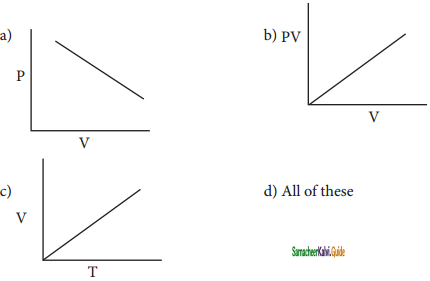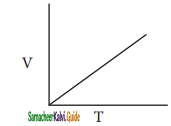Question 25.
25g of each of the following gases are taken at 27°C and 600 mm Hg pressure. Which of these will have the least volume?
(a) HBr
(b) HCl
(c) HF
(d) HI
(d) HIQuestion 26.
State Boyle’s law.
At a given temperature the volume occupied by a fixed mass of a gas is inversely proportional to its pressure.
Mathematically, the Boyle’s law can be written as
V ∝ $$\frac{1}{P}$$     …………..(1)
(T and n are fixed, T-temperature, n-number of moles)
V = k × $$\frac{1}{P}$$ …………(2)
k – proportionality constant
PV = k (at constant temperature and mass)

Question 27.
A balloon filled with air at room temperature and cooled to a much lower temperature can be used as a model for Charle’s law.
Yes, a balloon filled with air at room temperature and cooled to a much lower temperature can be used as a model for Charle’s law. Volume of balloon decrease when the temperature reduced from room temperature to low temperature. When cooled, the kinetic energy of the gas molecules decreases, so that the volume of the balloon also decreases.

Question 28.
Name two items that can serve as a model for ‘Gay Lusaac’ law and explain.
Firing a bullet:
When gunpowder burns, it creates; a significant amount of superheated gas. The high pressure of the hot gas behind the bullet forces it out, of the barrel of the gun.
Heating food in an oven:
When you keep food in an oven for heating, the air inside the oven is heated, thus pressurized.

Question 29.
Give the mathematical expression that relates gas volume and moles. Describe in words what j the mathematical expression means.
The mathematical expression that relates gas volume and moles is Avogadro’s hypothesis. It may be expressed as
V ∝ n,
$$\frac{V_{1}}{n_{1}}=\frac{V_{2}}{n_{2}}$$ = constant
where V1 and n1 are the volume and number of moles of a gas and V2 and n2 are a different set of values of volume and number of moles of the same gas at same temperature and pressure.Question 30.
What are ideal gases? In what way real gases differ from ideal gases?
The kinetic theory of gases which is the basis for the gas equation (PV = nRT), assumes that the individual gas molecules occupy negligible volume when j compared to the total volume of the gas and there is no attractive force between the gas molecules. Gases whose behaviour is consistent with these assumptions under all conditions are called ideal gases.

But in practice both these assumptions are not valid under all conditions. For example, the fact that gases can be liquefied shows that the attractive force exists among molecules. Hence, there is no gas which behaves ideally under all conditions. The non-ideal gases are called real gases. The real gases f tend to approach the ideal behaviour under certain conditions.

Question 31.
Can a Vander Waals gas with a = 0 be liquefied? Explain.
If the vander Waals constant (a) = 0 for a gas, then it behaves ideally, (i.e.,) there is no intermolecular forces of attraction. So it cannot be liquefied. Moreover,
Pc = $$\frac{a}{27 b^{2}}$$
If a = 0, then Pc = 0; therefore it cannot he liquefied.

Question 32.
Suppose there is a tiny sticky area on the wall of a container of gas. Molecules hitting this area stick there permanently. Is the pressure greater or less than on the ordinary area of walls?
Gaseous pressure is developed by the continuous bombardment of the molecules of the gas among themselves and also with the walls of the container. When the molecules hit the sticky area of the container, the number of molecules decreases and hence, the pressure decreases. Therefore, pressure is less than the ordinary area of walls.

Question 33.
Explain the following observations
(a) Aerated water bottles are kept under water during summer
(b) Liquid ammonia bottle is cooled before opening the seal
(c) The tyre of an automobile is inflated to slightly lesser pressure in summer than in winter
(d) The size of a weather balloon becomes larger and larger as it ascends up into larger altitude.
(a) Aerated water bottles contains excess dissolved oxygen and minerals which dissolved under certain pressure and if this pressure suddenly decrease due to change in atmospheric pressure, the bottle will certainly burst with decrease the amount of dissolved oxygen in water , this tends to change the aerated water into normal-water.

(b) The vapour pressure of ammonia at room temperature is very high and hence the ammonia will evaporate unless the vapour pressure is decreased. On cooling the vapour pressure decreases so that the liquid remains in the same state. Hence, the bottle is cooled ’ before opening.

(c) In Summer due to hot weather condition, the air inside the tyre expands to large volume due to heat as compared to winter therefore inflated to lesser pressure in summer.

(d) As we move to higher altitude, the atmospheric pressure decreases, therefore balloon can J easily expand to large volume.

Question 34.
Give suitable explanation for the following facts about gases.
(a) Gases don’t settle at the bottom of a container.
According to kinetic theory, gas molecules are moving continuous at random. Hence, they do not settle at the bottom of a container.

(b) Gases diffuse through all the space available to them.
Gases have a tendency to occupy all the available space. The gas molecules migrate from region of higher concentration to a region of lowrer concentration. Hence, gases diffuse through all the space available to them.Question 35.
Suggest why there is no hydrogen in our atmosphere. Why does the moon have no atmosphere?
Hydrogen has tendency to combine with oxygen to from water vapour. Hence, the presence of hydrogen is negligible in the atmosphere. The gravitational pull in the moon is very less and hence, there is no atmosphere in the moon.

Question 36.
Explain whether a gas approaches ideal behavior or deviates from ideal behaviour if
(a) it is compressed to a smaller volume at f constant pressure.
When the gas is compressed to a smaller volume, the compressibility factor (Z) decreases. Hence, the gas deviates from ideal behavior

(b) the temperature is raised at while keeping the volume constant.
When the temperature is increased, the compressibility factor approaches unity. Hence, the gas behaves ideally.

(c) More gas is introduced into the same volume and at the same temperature.
When more gas is introduced into a container of the same volume and at the same temperature, the compressibility factor tend to unity. Hence, the gas behaves ideally.

Question 37.
Which of the following gases would you expect to deviate from ideal behavior under conditions of low temperature F2, Cl2 or Br2? Explain.
Bromine has greater tendency to deviate from ideal behavior at low temperature. The compressibility factor tends to deviate from unity for bromine.

Question 38.
Distinguish between diffusion and effusion.

 Diffusion Effusion 1. The property of gas which involves the movement of the gas molecules through another gases is called diffusion. It is the property in which a gas escapes from a container through a very small hole. 2. It is the ability of gases to mix with each other It is ability of gas to travel through a small hole. 3. The rate of diffusion of a gas depends on how fast the gas molecules are moving The rate that this happens depends on how many gas molecules “collide” with the pore. 4. e.g., Smell of perfume diffuses into air e.g., Air escaping slowly through the pinhole in a tire.

Question 39.
Aerosol cans carry clear warning of heating of the can. Why?
If aerosol cans are heated, then they will produce more vapour inside the can, which will make the pressure rise very quickly. The rises of temperature can double the pressure inside. Even though the cans are tested, they will burst if the pressure goes up too far. A bursting can could be dangerous.Question 40.
When the driver of an automobile applies brake, the passengers are pushed toward the front of the car but a helium balloon is pushed toward back of the car. Upon forward acceleration the passengers are pushed toward the front of the car. Why?
Helium floats because it is buoyant; its molecules are lighter than the nitrogen and oxygen molecules of our atmosphere and so they rise above it. In the car, it’s the air molecules that are actually getting pulled and pushed around by gravity as the result of the accelerating frame.

That means it moves in a direction opposite to the force on the surrounding air. Normally, air is pulled downwards due to gravity, which pushes the balloon upwards. In this case, the surrounding air in the car is pulled forward by the deceleration of the car, which pushes the helium balloon backwards.

Question 41.
Would it be easier to drink water with a straw on the top of Mount Everest?
It would be harder on the top of the mountain; because the external pressure pushing on the liquid to force it up the straw is less.

Question 42.
Write the Van der Waals equation for a real gas. Explain the correction term for pressure and Volume.
Vander Waals equation for a real gas is given by
(P + $$\frac{a n^{2}}{V^{2}}$$)(V – nb) = nRT
Pressure Correction:
The pressure of a gas is directly proportional to the force created by the bombardment of molecules on the walls of the container. The speed of a molecule moving towards the wall of the container is reduced by the attractive forces exerted by its neighbours. Hence, the measured gas pressure is lower than the ideal pressure of the gas. Hence, van der Waals introduced a correction term to this effect.
Where n is the number of moles of gas and V is the volume of the container
⇒ P ∝ $$\frac{n^{2}}{V^{2}}$$
⇒ P = $$\frac{a n^{2}}{V^{2}}$$
Where a is proportionality constant and depends on the nature of gas
Therefore,
P = P + $$\frac{a n^{2}}{V^{2}}$$

Volume Correction:
As every individual molecule of a gas occupies a certain volume, the actual volume is less than the volume of the container, V. Van der Waals introduced a correction factor V to this effect. Let us calculate the correction term by considering gas molecules as spheres.
V = excluded volume
Excluded volume for two molecules = $$\frac{4}{3}$$ π(2r)3 = 8Vm
where Vm is a volume of a single molecule.
Excluded volume for single molecule = $$\frac{8 V_{m}}{2}$$ = 4Vm
Excluded volume for n molecule
= n(4Vm) = nb
Where b is van der waals constant which is equal to 4Vm
⇒ V’ = nb
Videal = V – nb
Replacing the corrected pressure and volume in the ideal gas equation PV = nRT we get the van der Waals equation of state for real gases as below,
(P + $$\frac{a n^{2}}{V^{2}}$$)(V – nb) = nRT
The constants a and b are van der Waals constants and their values vary with the nature of the gas.Question 43.
Derive the values of van der Vaals equation constants in terms of critical constants.
The Van der walls equation for n moles is
(p + $$\frac{a n^{2}}{V^{2}}$$)(V – nb) = nRT ……… (1)
For 1 mole
(P + $$\frac{n^{2}}{V^{2}}$$) (V – b) = RT ……………(2)

From the equation we can derive the values of critical constants Pc, Vc, and Tc in terms of a and b, the van der Waals constants, On expanding the above equation,
PV + $$\frac{a}{V}$$ – Pb – $$\frac{a b}{V^{2}}$$ – RT = 0 ………….(3)

Multiply equation (3) by $$\frac{V^{2}}{P}$$
$$\frac{V^{2}}{P}$$ (PV +$$\frac{a}{V}$$ – pb – $$\frac{a b}{V^{2}}$$ – RT) = 0

V3 + $$\frac{a}{P}$$V – bV2 – $$\frac{a b}{P}$$ – $$\frac{R T V^{2}}{P}$$ = 0 ………..(4)
When the above equation is rearranged in powers of V

V3 – [$$\frac{R T}{P}$$ + b]V2 + $$\frac{a}{P}$$V – $$\frac{a b}{P}$$ = 0 ……………..(5)

The equation (5) is a cubic equation in V. On solving this equation,
we will get three solutions. At the critical point all these three solutions of V are equal to the critical volume Vc. The pressure and temperature becomes Pc and Tc respectively
V = Vc
V – Vc = 0
(V – Vc)3 = 0
V3 – 3VcV2 + 3Vc2V – Vc3 = 0 …………..(6)
As equation (5) is identical with equation (6), we can equate the coefficients of V2, V and constant terms in (5) and (6).
-3VcV2 = –[$$\frac{R T_{c}}{P_{c}}$$ + b] V2

3Vc = $$\frac{R T_{c}}{P_{c}}$$ + b ………………(7)
3Vc2 = $$\frac{a}{P_{c}}$$ ……………(8)
Vc3 = $$\frac{ab}{P_{c}}$$ …………….(9)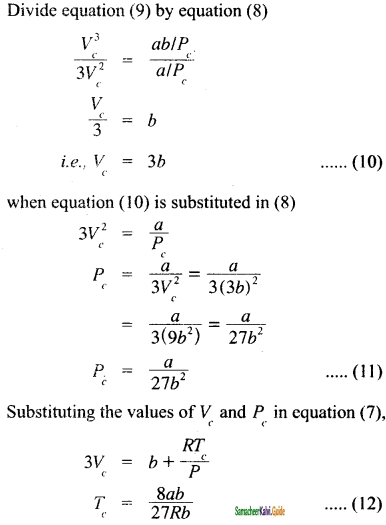The critical constants can be calculated using the values of van der waals constant of a gas and vice versa.
a = 3Vc2 Pc and
b = $$\frac{V_{c}}{3}$$

Question 44.
Why do astronauts have to wear protective suits when they are on the surface of moon?
Astronauts must wear spacesuits whenever they leave a spacecraft and are exposed to the environment of moon. In moon, there is no air to breath and no air pressure. Moon is extremely cold and filled with dangerous radiation. Without protection, an astronaut would quickly die in space. Spacesuits are specially designed to protect astronauts from the cold, radiation and low pressure in space. They also provide air to breathe. Wearing a spacesuit allows an astronaut to survive and work in moon.

Question 45.
When ammonia combines with HCl, NH4Cl is formed as white dense fumes. Why do more fumes appear near HCl?
HCl and NH4Cl molecules diffuse through the air towards each other. When they meet, they reactto
form a white powder called ammonium chloride, NH4Cl.
HCl(g) + NH3(g) ⇌ NHC1

Hydrogen chloride + ammonia ⇌ ammonium chloride.
The ring of white powder is closer to the HCl than
the NH3. This is because the NH3 molecules are lighter (smaller) and have diffùsed more quickly through the air in the tube.Question 46.
A sample of gas at 15°C at 1 atm. has a volume of 2.58 dm3. When the temperature is raised to 38°C at 1 atm does the volume of the gas Increase? If so, calculate the final volume.
T1 = 15°C + 273;
T2 = 38 + 273
T1 = 228;
T2 = 311K
V1 = 2.58dm3;
V2 = ?
(P = 1 atom constant)
$$\frac{V_{1}}{T_{1}}=\frac{V_{2}}{T_{2}}$$

V2 = $$\left[\frac{V_{1}}{T_{1}}\right]$$ × T2

= $$\frac{2.58 d m^{3}}{288 K}$$ × 311K
V2 = 2.78 dm3 i.e, volume increased from 2.58 dm3 to 2.78 dm3

Question 47.
Of two samples of nitrogen gas, sample A contains 1.5 moles of nitrogen In a vessel of volume of 37.6 dm3 at 298K, and the sample B Is in a vessel of volume 16.5 dm3 at 298K. Calculate the number of moles in sample B.
nA = 1.5mol; nB = ?
VA = 37.6 dm3; VB = 16.5 dm3
(T = 298K constant)
$$\frac{V_{A}}{n_{A}}=\frac{V_{B}}{n_{B}}$$

nA = $$\left[\frac{n_{A}}{n_{B}}\right] V_{B}$$

Question 48.
Sulphur hexafluoride Is a colourless, odourless gas; calculate the pressure exerted by 1.82 moles of the gas In a steel vessel of volume 5.43 dm3 at 69.5°C, assumIng Ideal gas behaviour.
n = 1.82 mole
V = 5.43 dm3
T = 69.5 + 273 = 342.5
P =?
PV = nRT
P = nRT/V
P =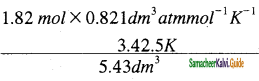P = 94.25 atm

Question 49.
Argon is an Inert gas used In light bulbs to retard the vaporization of the tungsten filament. A certain light bulb containing argon at 1.2 atm and 18°C Is heated to 85°C at constant volume. Calculate its final pressure in atm.
P1 = 1.2 atm
T1 = 180°C + 273 = 291 K
T2 = 850°C + 273 = 358 K
P2 =?
$$\frac{P_{1}}{T_{1}}=\frac{P_{2}}{T_{2}}$$

P2 = $$\left[\frac{P_{1}}{T_{1}}\right] \times T_{2}$$

P2 = $$\frac{1.2 a t m}{291 K}$$ × 358 K
P2 = 1.48 atmQuestion 50.
A small bubble rises from the bottom of a lake where the temperature and pressure are 6°C and 4 atm. to the water surface, where the temperature is 25°C and pressure Is I arm. Calculate the final volume in (mL) of the bubble, If its initial volume 1.5 mL.
T1 = 6°C + 273 = 279 K
P1 = 4 atm; V2 = 1.5 ml
T2 = 25°C + 273 = 298 K
P2 = 1 atm; V2 =?
$$\frac{P_{1} V_{1}}{T_{1}}=\frac{P_{2} V_{2}}{T_{2}}$$

= $$\frac{4 a t m \times 1.5 m l \times 298 K}{279 K \times 1 a t m}$$ = 6.41 mol

Question 51.
Hydrochloric acid Is treated with a metal to produce hydrogen gas. Suppose a student carries out this reaction and collects a volume of 154.4 × 10-3 dm3 of a gas at a pressure of 742 mm of Hg at a temperature of 298 K. What mass of hydrogen gas (in mg) did the student collect?
V = 154.4 × 10-3 dm3
P = 742 mm of Hg
T = 298K;
m =?
m = $$\frac{P V}{R T}$$

= $$\frac{742 m m H g \times 154.4 \times 10^{-3} L}{62 m m H g L K^{-1} m o l^{-1} \times 298 K}$$

n = $$\frac{\text { Mass }}{\text { Molar Mass }}$$

Mass = n × Molar Mass
= 0.0006 × 2.016
= 0.0121 g = 12.1 mg

Question 52.
It takes 192 sec for an unknown gas to diffuse through a porous wall and 84 sec for N2 gas to effuse at the same temperature and pressure. What Is the molar mass of the unknowa gas?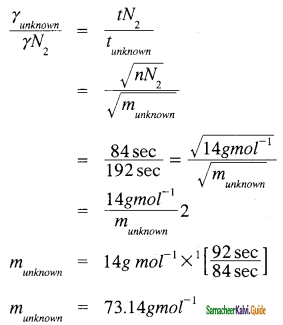Question 53.
A tank contains a mixture of 52.5 g of oxygen and 65.1 g of CO2 at 300 K the total pressure In the tanks Is 9.21 atm. calculate the partial pressure (in atm.) of each gas in the mixture.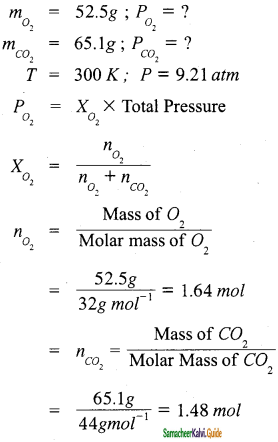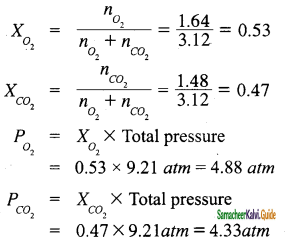Question 54.
A combustible gas is stored in a metal tank at a pressure of 2.98 atm at 25°C. The tank can withstand a maximum pressure of 12 atm after which It will explode. The building in which the tank has been stored catches fire. Now predict whether the tank will blow up first or start melting? (Melting point of the metal 1100 K).
Pressure of the gas in the tank at its melting point
T = 298 K;
P1 = 2.98 atom;
T2 = 1100 K;
P2 =?
$$\frac{P_{1} P_{2}}{T_{1} T_{2}}$$ =????
⇒ P2 = $$\frac{P}{T_{1}}$$ × T2
= $$\frac{2.98 \text { atm }}{298 K}$$ × 1100 K = 11 atm

At 1100 K the pressure of the gas inside the tank will become 11 atm. Given that tank can withstand a maximum pressure of 12 atm, the tank will start melting first.Question 1.
The approximate volume percentage of nitrogen and oxygen in the atmosphere of air are _______ and ______ respectively.
(a) 21, 68
(b) 21, 78
(c) 78, 2
(d) 80, 21
(c) 78, 2

Question 2.
The SI unit of pressure is
(a) pascal
(b) atmosphere
(c) bar
(d) torr
(a) pascal

Question 3.
The compound widely used in the refrigerator as coolant is
(a) Freon-2
(b) Freon-12
(c) Freon-13
(d) Freon-14
(b) Freon-12

Question 4.
“For a fixed mass of a gas at constant pressure, the volume is directly proportional to its temperature”. This statement is
(a) Boyle’s law
(b) Gay-Lussac law
(d) Charle’s law
(d) Charle’s law

Question 5.
Hydrogen is placed in the ______ of the periodic table.
(a) Group -1
(b) group-17
(c) group-18
(d) group-2
(a) Group -1Question 6.
The plot of the volume of the gas against its temperature at a given pressure is called
(a) isotone
(b) isobar
(c) isomer
(d) isotactic
(b) isobar

Question 7.
At constant temperature for a given mass, for each degree rise in temperature, all gases expand by of their volume at 0°C.
(a) 273
(b) 298
(c) $$\frac{1}{273}$$

(d) $$\frac{1}{298}$$
(c) $$\frac{1}{273}$$

Question 8.
The precise value of temperature at which the volume of the gas becomes zero is
(a) -273.15 °C
(b) -273 °C
(c) -298.15°C
(d) -298 °C
(a) -273.15 °C

Question 9.
‘At constant volume, the pressure of a fixed mass of a gas is directly proportional to temperature”. This statement is
(a) Charle’s law
(b) Boyle’s law
(c) Gay-Lussac’s law
(d) Dalton’s law
(c) Gay-Lussac’s law

Question 10.
The value of gas constant, R, in terms of JK-1 mol-1 is
(a) 8.314
(b) 4.184
(c) 0.0821
(d) 1.987
(a) 8.314Question 11.
A mixture of gases containing 4 mole of hydrogen and 6 mole of oxygen. The partial pressure of hydrogen, if the total pressure is 5 atm, is
(a) 10 atm
(b) 0.4 atm
(c) 20 atm
(d) 2 atm
(d) 2 atm

Question 12.
A mixture of gases containing 1 mole of He, 4 mole of Ne and 5 mole of Xe. The correct order of partial pressure of the gases, if the total pressure is 10 atm is
(a) Xe < Ne < He
(b) He < Ne < Xe
(c) Xe < Ne < He
(d) He < Xe < Ne
(b) He < Ne < Xe

Question 13.
The property of a gas which involves the movement of the gas molecules through another gases is called
(a) effusion
(b) dissolution
(c) difusion
(d) expansion
(c) difusion

Question 14.
The process in which agas escapes from a container through a very small hole is called
(a) diffusion
(b) effusion
(c) occlusion
(d) dilution
(a) diffusion

Question 15.
The rate of diffusion of a gas is inversely proportional to the
(a) square of molar mass
(b) square root of density
(c) square root of molar mass
(d) square of density
(c) square root of molar massQuestion 16.
An unknown gas X diffuses at a rate of 2 times of oxygen at the same temperature and pressure. The molar mass (in g mol-1) of the gas ‘X is (Molar mass of oxygen is 32 g mol-1)
(a) 8
(b) 16
(c) 20
(d)12
(a) 8

Question 17.
The deviation of real gases from ideal behavior is measured in terms of
(a) expansivity factor
(b) molar mass
(c) pressure
(d) compressibility factor
(d) compressibility factor

Question 18.
The gases which deviate from ideal behavior at
(a) low temperature and high pressure
(b) high temperature and low pressure
(c) low temperature and low pressure
(d) high temperature and high pressure
(b) high temperature and low pressure

Question 19.
The temperature at which a real gas obeys ideal gas law over an appreciable range of pressure is called temperature
(a) inversion
(b) ideal
(c) Boyle
(d) reversible
(c) Boyle

Question 20.
Ideal gas equation for ‘n’ moles is
(a) $$\frac{P}{R}=\frac{n T}{V}$$

(b) PV = $$\frac{n R}{T}$$

(c) $$\frac{V}{T}=\frac{n P}{R}$$

(d) $$\frac{P}{T}=\frac{n R}{V}$$
(d) $$\frac{P}{T}=\frac{n R}{V}$$Question 21.
The pressure correction introduced by Van der Waals is,
Pideal =
(a) P + $$\frac{a V^{2}}{n^{2}}$$

(b) P + $$\frac{a n}{V^{2}}$$

(c) P + $$\frac{a n^{2}}{V^{2}}$$

(d) P + $$\frac{a V}{n^{2}}$$
(c) P + $$\frac{a n^{2}}{V^{2}}$$

Question 22.
The volume correction introduced by Van der Waals is, Videal =
(a) V – nb
(b) V + nb
(c) $$\frac{V}{n b}$$
(d) b – nV
(b) V + nb

Question 23.
Van der waals equation for one mole of a gas is
(a) (P + $$\frac{a}{V}$$)(V-b) = RT
(b) (P – $$\frac{a}{V^{2}}$$)(V + -b) = RT
(c) (P + $$\frac{a}{V^{2}}$$)(V – b) = RT
(d) (P + $$\frac{a}{V}$$)(V + b) = RT
(c) (P + $$\frac{a}{V^{2}}$$)(V – b) = RT

Question 24.
The unit of Van der Waals constant V is
(a) atm lit mol-1
(b) atm lit2 mol-2
(c) atm lit-2 mol2
(d) atm lit-1 mol2
(b) atm lit2 mol-2

Question 25.
The unit of Van der waals constant ‘b’ is
(a) lit mol-1
(b) lit mol
(c) atm lit mol-1
(d) atm lif-1 mol-2
(a) lit mol-1Question 26.
The temperature above which a gas cannot be liquefied even at high pressure is called is ________ temperature.
(a) ideal
(b) inversion
(c) critical
(d) real
(c) critical

Question 27.
The gas used in pressure-volume isotherm study of Andrew’s experiment is
(a) N2
(b) H2S
(c) NH3
(d) CO2
(d) CO2

Question 28.
Which of the following gas has highest critical temperature?
(a) NH3
(b) CO2
(c) N2
(d) CH4
(a) NH3

Question 29.
The relationship between critical volume and Vander waals constant is
(a) Vc =$$\frac{a}{R b}$$
(b) Vc = 3b
(c) Vc = $$\frac{8 a}{27 R b}$$
(d) Vc = 8b
(b) Vc = 3b

Question 30.
The temperature below which a gas obeys Joule-Thomson effect is called ______ temperature.
(a) critical
(b) Inversion
(c) ideal
(d) real
(b) InversionQuestion 31.
In the equation PV = nRT, which one cannot be numerically equal to R?
(a) 8.31 × 107 ergs K-1 mol-1
(b) 8.31 × 107 dynes cm K-1 mol-1
(c) 8.317J K-1 mol-1
(d) 8.317L atm K-1 mol-1
(d) 8.317L atm K-1 mol-1

Question 32.
A sample of a given mass of gas at constant temperature occupies a volume of 95 cm3 under a pressure of 10.13 × 104 Nm-2. At the same temperature, its volume at pressure of 10.13 × 104 Vm-2 is
(a) 190 cm3
(b) 93 cm3
(c) 46.5 cm3
(d) 4.75 cm3
(b) 93 cm3

Question 33.
The number of moles of H2 in 0.224 L of hydrogen gas at STP (273 K, 1 atm) assuming ideal gas behavior is
(a) 1
(b) 0.1
(c) 0.01
(d) 0.001
(c) 0.01

Question 34.
Use of hot air balloons in sports and meteorological observations is an application of
(a) Boyle’s law
(b) Newton’s law
(c) Kelvin’s law
(d) Charle’s law
(d) Charle’s law

Question 35.
To what temperature must a neon gas sample be heated to double its pressure, if the initial volume of gas at 75°C is decreased by 15.0% by cooling the gas?
(a) 319°C
(b) 592°C
(c) 128°C
(d) 60°C
(a) 319°CQuestion 36.
7.0 g of a gas at 300K and 1 atm occupies a volume of 4.1 litre. What is the molecular mass of the gas?
(a) 42
(b) 38.24
(c) 14.5
(d) 46.5
(a) 42

Question 37.
If most probable velocity is represented by a and fraction possessing it by / then with increase in temperature which one of the following is correct?
(a) α increases, f decreases
(b) α decreases, f increases
(c) Both α and f decrease
(d) Both α and f increase
(a) α increases, f decreases

Question 38.
The rate of diffusion of gases A and B of molecular weight 36 and 64 are in the ratio
(a) 9 : 16
(b) 4 : 3
(c) 3 : 4
(d) 16 : 9
(b) 4 : 3

Question 39.
To which of the following gaseous mixtures Dalton’s law is not applicable?
(a) Ne + He + SO2
(b) NH3 + HCl + HBr
(c) O2 + N2 +CO2
(d) N2 + H2 + O2
(b) NH3 + HCl + HBr

Question 40.
Which of the following is true about gaseous state?
(a) Thermal energy = molecular interaction
(b) Thermal energy >> molecular interaction
(c) Thermal energy << molecular interaction
(d) molecular forces >> those in liquids
(b) Thermal energy >> molecular interactionQuestion 41.
50 mL of gas A effuses through a pinhole in 146 seconds. The same volume of CO2 under identical condition effuses in 115 seconds. The molar mass of A is
(a) 44
(b) 35.5
(c) 71
(d) None of these
(c) 71

Question 42.
Gas deviates from ideal gas nature because molecules
(a) are colourless
(b) attract each other
(c) contain covalent bond
(d) show Brownian movement
(b) attract each other

Question 43.
Which of the following gases is expected to have largest value of van der Waals constant ‘a’?
(a) He
(b) H2
(c) NH3
(d) O2
(c) NH3

Question 44.
In van der Waals equation of state for a real gas, the term that accounts for intermolecular forces is
(a) Vm – b
(b) P + $$\frac{a}{V m^{2}}$$
(c) RT
(d) $$\frac{1}{R T}$$
(b) P + $$\frac{a}{V m^{2}}$$

Question 45.
What are the most favourable conditions to liquefy a gas?
(a) High temperature and low pressure
(b) Low temperature and high pressure
(c) High temperature and high pressure
(d) Low temperature and low pressure
(b) Low temperature and high pressureQuestion 46.
Which of the following has a non-linear relationship?
(a) P vs V
(b) P vs $$\frac{1}{V}$$
(c) both (a) and (b)
(d) none of these
(a) P vs V

Question 47.
Why is that the gases show ideal behavior when the volume occupied is large?
(a) So that the volume of the molecules can be neglected in comparison to it.
(b) So that the pressure is very high
(c) So that the Boyle temperature of the gas is constant
(d) all of these
(a) So that the volume of the molecules can be neglected in comparison to it.

Question 48.
At a given temperature, pressure of a gas obeying Van der Waals equation is
(a) less than that of an ideal gas
(b) more than that of an ideal gas
(c) more or less depending on the nature of gas
(d) equal to that of an ideal gas
(a) less than that of an ideal gas

Question 49.
NH3 gas is liquefied more easily than N2. Hence
(a) Van der Waals constant a and b of NH3 > that of N2
(b) van der Waals constants a and b of NH3 < that of N2
(c) a(NH3) > a(N2) but b(NH3) < b(N2)
(d) a(NH3) < a(N2) but b(NH3) > b(N2)
(c) a(NH3) > a(N2) but b(NH3) < b(N2)

Question 50.
Maximum deviation from ideal gas is expected from
(a) CH4(g)
(b) NH3(g)
(c) H2(g)
(d) N2(g)
(b) NH3(g)II. Very short question and answers (2 Marks):

Question 1.
Define Pressure? Give its unit?
Pressure is defined as force divided by the area to which the force is applied. The SI unit of pressure is pascal which is defined as 1 Newton per square meter (Nm-2).
Pressure = $$\frac{\text { Force }\left(\mathrm{N}(\text { or }) \mathrm{Kg} \mathrm{ms}^{-2}\right)}{\text {Area }\left(\mathrm{m}^{2}\right)}$$

Question 2.
State Gay Lussac ‘s law.
At constant volume, the pressure of a fixed mass of a gas is directly proportional to temperature.
P ∝ T or $$\frac{P}{T}$$ = constant K
If P1 and P2 are the pressures at temperatures T1 and T2, respectively, then from Gay Lussac’s law
$$\frac{P_{1}}{T_{1}}=\frac{P_{2}}{T_{2}}$$

Question 3.
State Dalton’s law of partial pressure.
The total pressure of a mixture of non-reacting gases is the sum of partial pressures of the gases present in the mixture” where the partial pressure of a component gas is the pressure that it would exert if it were present alone in the same volume and temperature. This is known as Dalton s law of partial pressures.
For a mixture containing three gases 1, 2, and 3 with partial pressures p1, p2, and p3 in a container with volume V, the total pressure Ptotal will be give by
Ptotal = p1 + p2 + p3

Question 4.
What is Compressibility factor?
The deviation of real gases from ideal behaviour is measured in terms of a ratio of PV to nRT. This is termed as compressibility factor. Mathematically,
Z = $$\frac{P V}{n R T}$$

Question 5.
Define Critical temperature (Tc) of a gas?
Critical temperature (Tc) of a gas is defined as the temperature above which it cannot be liquefied even at high pressure.Question 6.
How does cooling is produced in Adiabatic Process?
In Adiabatic process, cooling is produced by removing the magnetic property of magnetic material such as gadolinium sulphate. By this method, a temperature of 10-4 K i.e., as low as 0 K can be achieved.

Question 7.
How do you understand PV relationship?
The PV relationship can be understood as follows. The pressure is due to the force of the gas particles on the walls of the container. If a given amount of gas is compressed to half of its volume, the density is doubled and the number of particles hitting the unit area of the container will be doubled. Hence, the pressure would increase two fold.

Question 8.
Write a note on Consequence of Boyle’s law.
The pressure-density relationship can be derived from the Boyle’s law as shown below.
P1V1 = P2V2
P1 $$\frac{m}{d_{1}}$$ = P2 $$\frac{m}{d_{2}}$$
where “m” is the mass, d1 and d2 are the densities of gases at pressure P1 and P2.
$$\frac{P_{1}}{d_{1}}=\frac{P_{2}}{d_{2}}$$
In other words, the density of a gas is directly proportional to pressure.

Question 9.
A gas cylinder can withstand a pressure of 15 aim. The pressure of cylinder is measured 12 atm at 27°C. Upto which temperature limit the cylinder will not burst?
Cylinder will burst at that temperature when it attains the pressure of 15 atm
P1 = 12 atm
T = 27° C = (27 + 273)K = 300K
P2 = 15 atm; T = ?
$$\frac{P_{1}}{T_{1}}=\frac{P_{2}}{T_{2}}$$

T2 = $$\frac{15 \times 300}{12}$$ = 375 K
= (375 – 273)°C = 102°C

Question 10.
Write a note on application of Dalton’s law.
In a reaction involving the collection of gas by downward displacement of water, the pressure of dry vapor collected can be calculated using Dalton’s law.
Pdry gas collected = Ptotal – Pwatervapour
Pwatervapour has generally referred as aqueous tension and its values are available for air at various temperatures.Question 11.
State Charles law.
For a fixed mass of a gas at constant pressure, the volume is directly proportional to its temperature.
$$\frac{V}{T}$$ = constant (at constant pressure)

Question 12.
What happens when a balloon is moved from an ice cold water bath to a boiling water bath?
If a balloon is moved from an ice cold water bath to a boiling water bath, the temperature of the gas increases. As a result, the gas molecules inside the balloon move faster and gas expands. Hence, the volume increases.

Question 13.
Write notes on coefficient of expansion(α).
The relative increase in volume per °C (α) is equal to $$\frac{V}{V_{0} T}$$
Therefore, $$\frac{V}{V_{0} T}$$
TV = V0(αT + 1)
Charles found that the coefficient of expansion is approximately equal to 1/273. It means that at constant temperature for a given mass, for each degree rise in temperature, all gases expand by 1/273 of their volume at 0°C.

Question 14.
State Gay-Lussac law.
At constant volume, the pressure of a fixed mass of a gas is directly proportional to temperature.
P ∝ T (at constant volume)

Question 15.
Equal volumes of all gases under the same conditions of temperature and pressure contain equal number of molecules.Question 16.
Distinguish between diffusion and effusion.
The property of gas that involves the movement of the gas molecules through another gases is called diffusion. Effusion is another process in which a gas escapes from a container through a very small hole.

Question 17.
State Graham’s law of diffusion.
The rate of diffusion or effusion is inversely proportional to the square root of molar mass. This statement is called Graham’s law of diffusion/effusion.
Mathematically, rate of diffusion ∝ $$\frac{1}{M}$$

Question 18.
What is Boyle temperature?
The temperature at which a real gas obeys ideal gas law over an appreciable range of pressure is called Boyle temperature or Boyle point.III. Short Question and Answers (3 Marks):

Question 1.
Write notes on Boyle’s point.
The temperature at which a real gas obeys ideal gas law over an appreciable range of pressure is called Boyle temperature or Boyle point. The Boyle point varies with the nature of the gas. Above the Boyle point, for real gases, Z > 1, i.e., the real gases show positive deviation. Below the Boyle point, the real gases first show a decrease for Z, reaches a minimum and then increases with the increase in pressure.

Question 2.
What are the different methods of liquefaction of gases?
There are different methods used for liquefaction of gases:

1. In Linde’s method, Joule-Thomson effect is used to get liquid air or any other gas.
2. In Claude’s process, the gas is allowed to perform mechanical work in addition to Joule-Thomson effect so that more cooling is produced.
3. In Adiabatic process, cooling is produced by removing the magnetic property of magnetic material such as gadolinium sulphate. By this method, a temperature of 10-4 K i.e., as low as 0 K can be achieved.

Question 3.
48 litre of dry N2 is passed through 36g of H2O at 27°C and this results In a loss of 1.20 g of water. Find the vapour pressure of water?
Water loss is observed because of escape of water molecules with N2 gas. These water vapour occupy the volume of N2 gas i.e., 48 litres.
Using,
PV = $$\frac{m}{M}$$RT;
V = 48L
m = 1.2g;
M = 18u;
R = 0.082 L atm K-1mol-1
T = 27 + 273 = 300 K
P = $$\frac{1.2}{18} \times \frac{0.0821 \times 300}{48}$$ = 0.034 atmQuestion 4.
A flask of capacity one litre is heated from 25°C to 35°C. What volume of air will escape from the flask?
Applying, $$\frac{V_{1}}{T_{1}}=\frac{V_{2}}{T_{2}}$$
V1 = 1L;
T1 = 25 + 273 = 298 K
V2 = ?
T2 = 35 + 273 = 308 K
V2 = $$\frac{1}{298}$$ × 308 = 1.033 L
Capacity of Flask = 1 L
So, volume of air escaped = 1.033 – 1 = 0.033 L = 33 mL

Question 5.
Discuss the graphical representation of Boyle’s law.
Boyle’s law is applicable to all gases regardless of their chemical identity (provided the pressure is low). Therefore, for a given mass of a gas under two different sets of conditions at constant temperature we can write,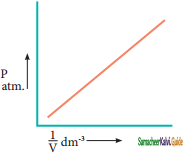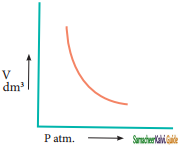Question 6.
A certain gas takes three times as long to effuse out as helium. Find its molecular mass.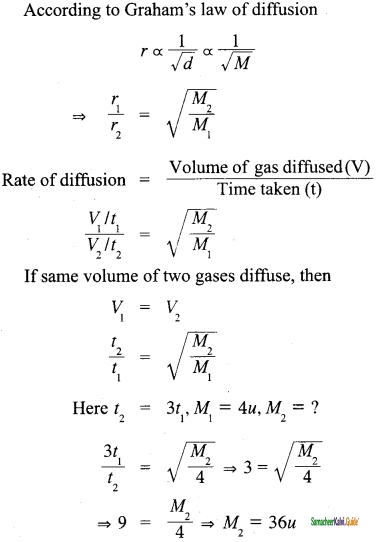Question 7.
The vapour pressure of water at 80°C is 355.5 mm of Hg. A 100 mL vessel contains water saturated with O2 at 80°C, the total pressure being 760 mm of Hg. The contents of the vessel were pumped into a 50 mL vessel at the same temperature. What is the partial pressure of O2?
Ptotal = PH2O + PO2
PO2 = 760 – 355.5 = 404.5
When the contents were pumped into 50 mL vessel
P1 = 404.5
V1 = 100 mL
P2 =?
V2 = 50 mL
P1V1 = P2V2
P2 = $$\frac{404.5 \times 100}{50}$$ = 809 mm
Thus, PO2 in 50 mL vessel = 809 mmQuestion 8.
Write notes on compressibility factor for real gases.
The compressibility factor Z for real gases can be rewritten,
Z = PVreal / nRT …………..(1)
Videal = $$\frac{n R T}{P}$$ ……………(2)
substituting (2) in (1)
where Vreal is the molar volume of the real gas and Videal is the molar volume of it when it behaves ideally.

Question 9.
Derive ideal gas equation.
The gaseous state is described completely using the following four variables T, P, V, and n and their relationships were governed by the gas laws studied so far.
Boyle’s law V ∝ P1
Charles law V ∝ T
We can combine these equations into the following general equation that describes the physical behaviour of all gases.
V ∝ $$\frac{n T}{P}$$

V = $$\frac{n R T}{P}$$
where, R is the proportionality constant called universal gas constant.
The above equation can be rearranged to give the ideal gas equation
PV = nRT

Question 10.
State and explain Dalton’s law of partial pressure.
John Dalton stated that “the total pressure of a mixture of non-reacting gases is the sum of partial pressures of the gases present in the mixture” where the partial pressure of a component gas is the pressure that it would exert if it were present alone in the same volume and temperature. This is known as Dalton’s law of partial pressures, i.e., for a mixture containing three gases 1, 2, and 3 with partial pressures p1, p2, and p3 in a container with volume V, the total pressure Ptotal will be given by
P = p1 + p2 + p3IV. Long Question and Answers (5 Marks):

Question 1.
Derive Van der waals equation of state.
J.D.Van der Waals made the first mathematical analysis of real gases. His treatment provides us an interpretation of real gas behaviour at the molecular level. He modified the ideal gas equation PV = nRT by introducing two correction factors, namely, pressure correction and volume correction.

Pressure Correction:
The pressure of a gas is directly proportional to the force created by the bombardment of molecules on the walls of the container. The speed of a molecule moving towards the wall of the container is reduced by the attractive forces exerted by its neighbours. Hence, the measured gas pressure is lower than the ideal pressure of the gas. Hence, van der Waals introduced a correction term to this effect.

Van der Waals found out the forces of attraction experienced by a molecule near the wall are directly proportional to the square of the density of the gas.
P’ ∝ p2
p = $$\frac{n}{v}$$

where n is the number of moles of gas and V is the volume of the container
⇒ P’ = $$\frac{n^{2}}{V^{2}}$$
⇒ P’ = a$$\frac{n^{2}}{V^{2}}$$

where a is the proportionality constant and depends on the nature of gas.
Therefore, ideal P = P + a$$\frac{n^{2}}{V^{2}}$$

Volume Correction:
As every individual molecule of a gas occupies a certain volume, the actual volume is less than the volume of the container, V. Van der Waals introduced a correction factor V’ to this effect. Let us calculate the correction term by considering gas molecules as spheres.
V = excluded volume
Excluded volume for two molecules = $$\frac{4}{3}$$π(2r)3
= $$\left|\frac{-8}{(3 \pi)}\right|$$ = 8Vm

where Vm is a volume of a single molecule
Excluded volume for single-molecule = $$\frac{8 V_{m}}{2}$$ = 4Vm

Excluded volume for n molecule = n(4Vm) = nb
Where b is van der Waals constant which is equal to 4 Vm
=> V’ = nb
Videal = V nb

Replacing the corrected pressure and volume in the ideal gas equation PV = nRT we get the van der Waals equation of state for real gases as below,
(P + $$\frac{a n^{2}}{V}$$)(V – nb) = nRT
The constants a and b are van der Waals constants and their values vary with the nature of the gas. It is an approximate formula for the non-ideal gas.Question 2.
Derive the relationship between Van der Waals constants and critical constants.
The van der Waals equation for n moles is
(P + $$\frac{a n^{2}}{V}$$)(V – nb) = nRT
For 1 mole
(p + $$\frac{a}{V^{2}}$$)(V – b) = RT
From the equation we can derive the values of critical constants Pc, Vc, and Tc in terms of a and b, the van der Waals constants, On expanding the above equation
PV + $$\frac{a}{V}$$ – Pb – $$\frac{a b}{V^{2}}$$ – RT = 0

Multiply above equation by
$$\frac{V_{2}}{P}$$(PV + $$\frac{a}{V}$$ – Pb – $$\frac{a b}{V^{2}}$$ – RT) = 0

V3 + $$\frac{a V}{P}$$ + -bV2 – $$\frac{a b}{p}$$ – $$\frac{R T V^{2}}{P}$$ = 0

when the above equation is rearranged in powers of V

V3 $$\frac{R T}{P}$$ + bV2 $$\frac{a}{P}$$ V – $$\frac{a b}{P}$$ = 0.

The above equation is a cubic equation in V. On solving this equation,
we will get three solutions. At the critical point, all these three solutions of Vc are equal to the critical volume. The pressure and temperature becomes Pc and Tc respectively
i.e., V = Vc
V – Vc = 0
V – (Vc)3 = 0
V3 – 3VcV2 + 3VVc2 – Vc3 = 0.
As equation identical with equation above, we can equate the cocfficients of Vc, V and constant terms.

-3VcV2 = –$$\frac{R T_{C}}{P}$$ + bV2

3Vc = $$\frac{R T_{C}}{P_{C}}$$ + b ……….(1)

3Vc2 = $$\frac{a}{P_{C}}$$ …………..(2)

Vc3 = $$\frac{a b}{P_{C}}$$ …………..(3)

Divide equation (3) by equation (2)

$$\frac{V_{c}^{3}}{3 V_{C}^{2}}=\frac{a b / P_{C}}{a / P_{C}}$$

$$\frac{V_{C}}{3}$$ = b
i.e., Vc = 3b ……………(4)

when equation (4) is substituted in (2)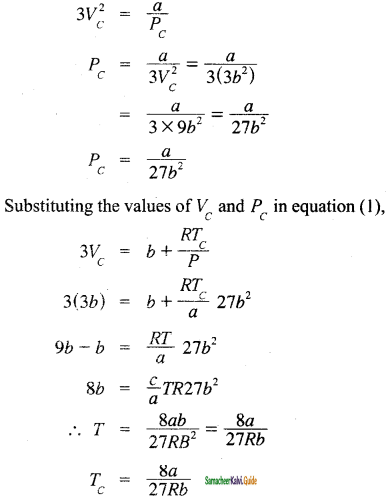The critical constants can be calculated using the values of vander walls constant of a gas and vice versa.

a = 3Vc2 Pc and b = $$\frac{V_{C}}{3}$$Question 3.
Explain Andrew’s isotherm for Carbon dioxide.
Thomas Andrew gave the first complete data on pressure-volume-temperature of a substance in the gaseous and liquid states. He plotted isotherms of carbon dioxide at different temperatures which is shown in Figure. From the plots we can infer the following.

At low temperature isotherms, for example, at 130°C as the pressure increases, the volume decreases along AB and is a gas until the point B is reached. At B, a liquid separates along the line BC, both the liquid and gas co-exist and the pressure remains constant. At C, the gas is completely converted into liquid. If the pressure is higher than at C, only the liquid is compressed so, there is no significant change in the volume. The successive isotherms shows similar trend with the shorter flat region. i.e., The volume range in which the liquid and gas coexist becomes shorter.

At the temperature of 31.1°C the length of the shorter portion is reduced to zero at point P. In other words, the CO2 gas is liquefied completely at this point. This temperature is known as the liquefaction temperature or critical temperature of CO3. At this point the pressure is 73 atm. Above this temperature, CO3 remains as a gas at all pressure values. It is then proved that many real gases behave in a similar manner to carbon dioxide.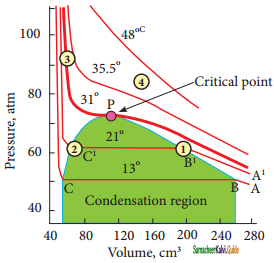Question 4.
Explain Boyle’s law experiment: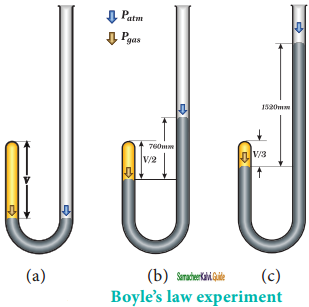V ∝ $$\frac{1}{P}$$ ……….(1)
V = k × $$\frac{1}{P}$$ ……….(2)